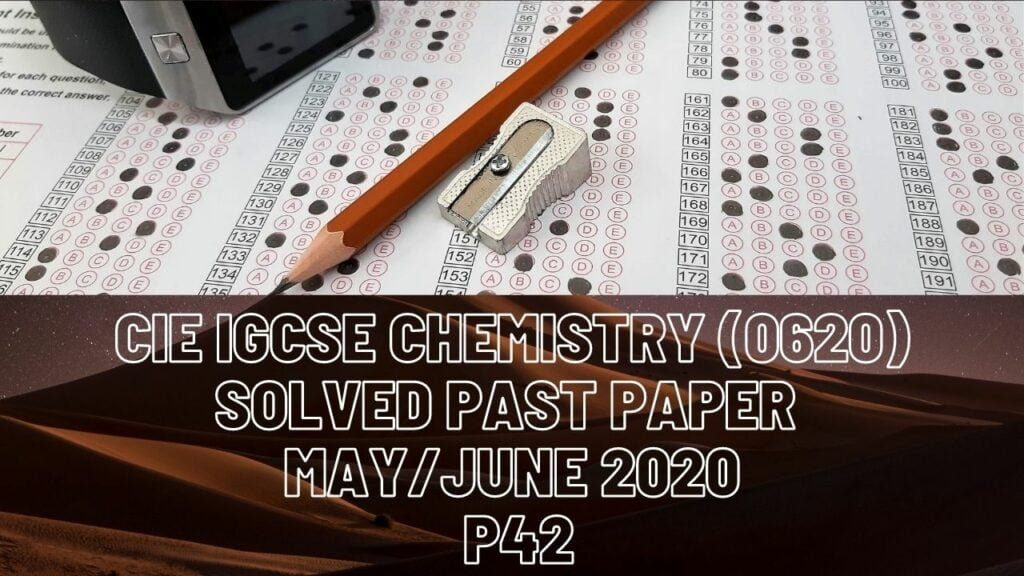IGCSE Chemistry### CIE IGCSE Chemistry Solved Past Paper May/June 2020 P42

1a(i) Condensation
(ii) Sublimation
(iii) Fractional distillation
(iv) Electrolysis
(v) Chromatography
b(i) N
(ii) O
(iii) Be
(iv) N
(v) Ne
(vi) Li

2a(i) Magnesium 2+ ion forms after magnesium loses 2 electrons from its outer most shell – fluoride ion forms after fluorine gains 1 electron.
(ii) MgF2
(iii) Heat until it is molten, ions can now move in this form and so conduct
electricity.
b) Carbon forms 2 single bonds with 2 fluorine atoms each, and 1 double
bond with oxygen
c(i) Strong ionic bonds need a lot of energy to be broken because forces of
attraction between oppositely charged ions are strong
(ii) Forces of attraction between molecules are weak(bonding in covalent
compounds)

3a(i) It is heated in air
(ii) 2SO2 + O2——-> 2SO3
450 degrees Celsius, Vanadium (V) Oxide
(iii) Rate of forward reaction is equal to rate of backward reaction, and
concentration of reactants and products remains the same
b) Concentrated sulfuric acid
c) H2S2O7 + H2O——-> 2H2SO4
d) 2-2
e) From purple to colourless
f) 2NH3 + H2SO4——-> (NH4)2SO4
g(i) Barium chloride
(ii) Ba2+ (aq)+ SO4(2-)(aq) —-> BaSO4(s)

4a) A substance that increases the rate of a chemical reaction without
being used up itself
b) Rate decreases, concentration of hydrogen peroxide decreases, collision frequency is reduced as a result of this. At the end all of hydrogen peroxide is used up, which stops the reaction.
d) Rate of reaction increases, because molecules gain kinetic energy, they move faster, collision frequency increases, more molecules have sufficient energy to react.
e) Moles = volume/molar volume
= (48/1000)/24
= 0.002 mol
2H2O2 —-> 1O2
So 0.002mol —> 0.002*2 = 0.004mol
Concentration = moles/volume
= 0.004/(25/1000)
= 0.16 mol/dm3
f) 2KCLO3——–>2KCL+3O2

5a) Breakdown of an ionic compounds when molten or in aqueous form by
passage of electricity
b) Graphite
c) 2H+ + 2e- —-> H2
d) Na+, H+, OH-, Cl-
e) After H+ and Cl- ions are removed from the solution, Na+ and OH- remain in solution forming sodium hydroxide

6a(i) Substitution
(ii) Ultraviolet light
(iii) Chlorine on carbon 1 and on carbon 2
b(i) Because 2 molecules join to make a product molecule

(ii) On the reactant side, the bonds broken are: C=C double bonds and a single Cl-Cl bond, on the reactant side, the new bonds formed are: 2Cl-Cl
And 1 C-C bond
Therefore change in energy =
((2612)+242) – ((2339)+347)= -171
c) Alkene, alcohol, carboxylic acids
(ii) Solution turns colourless from brown
Bubbles (acid + alkali)
d(i) Double bond opens up to allow extension of structure
(ii) Polyester